Ex 7.7

Chapter 7 Class 12 Integrals
Serial order wise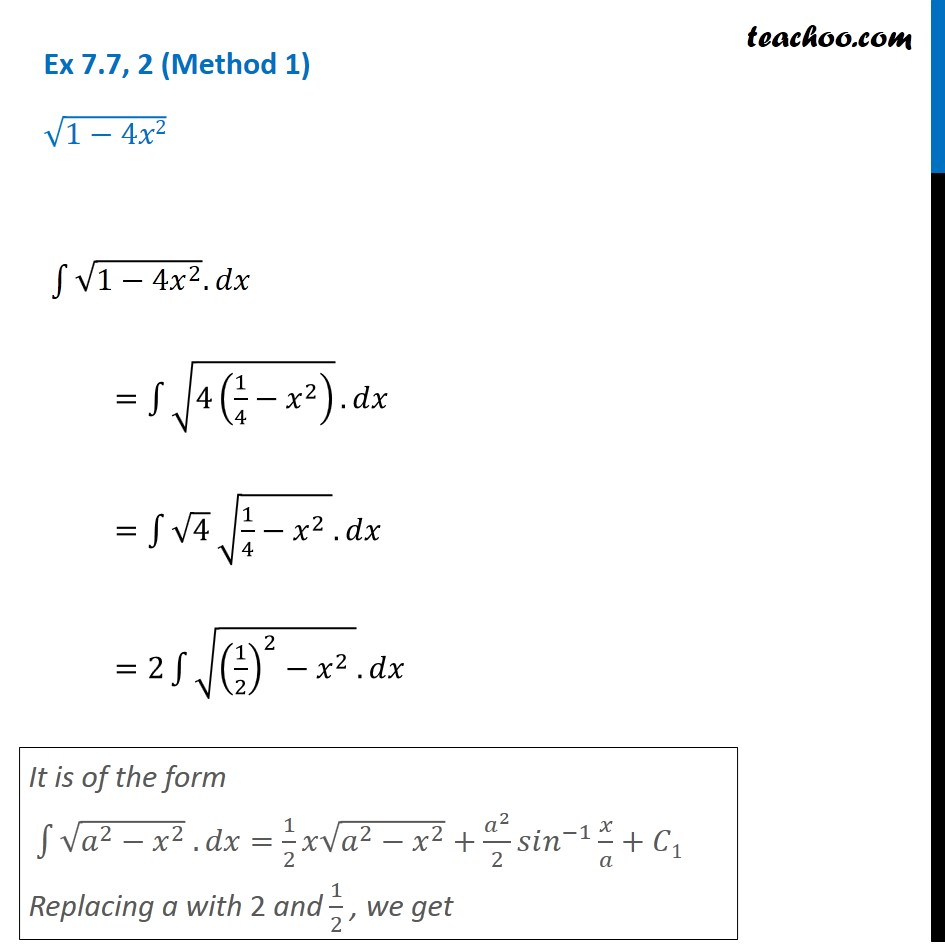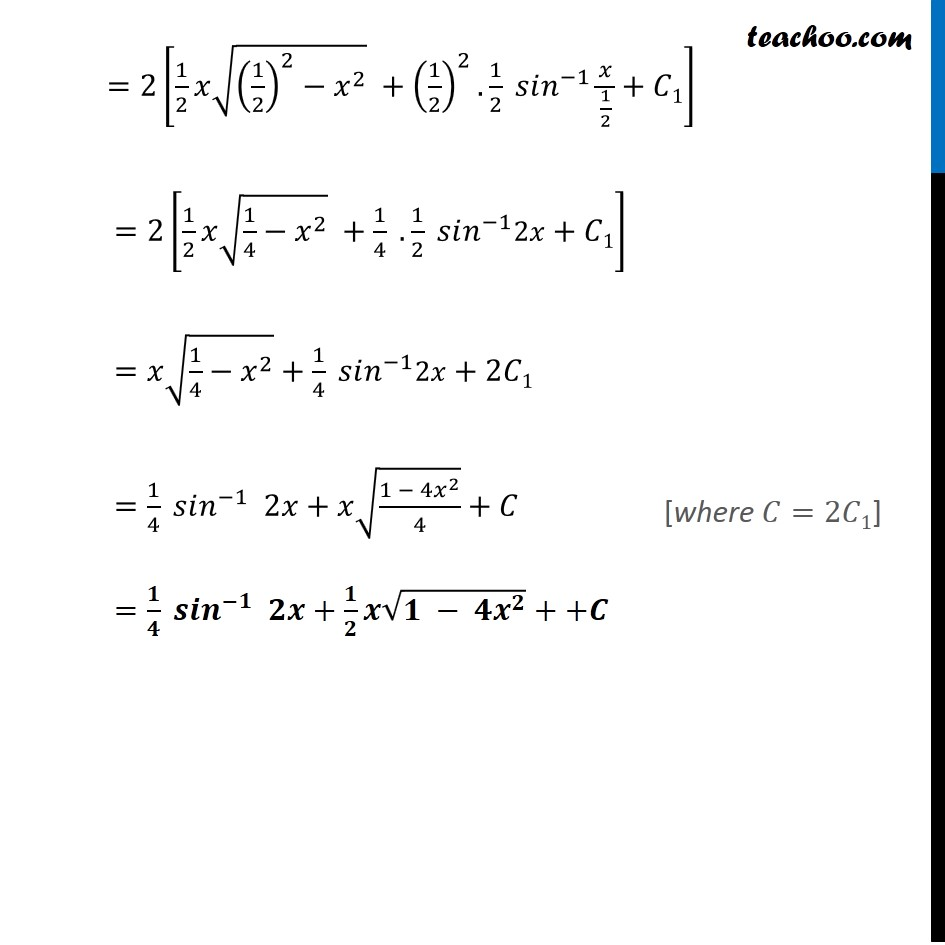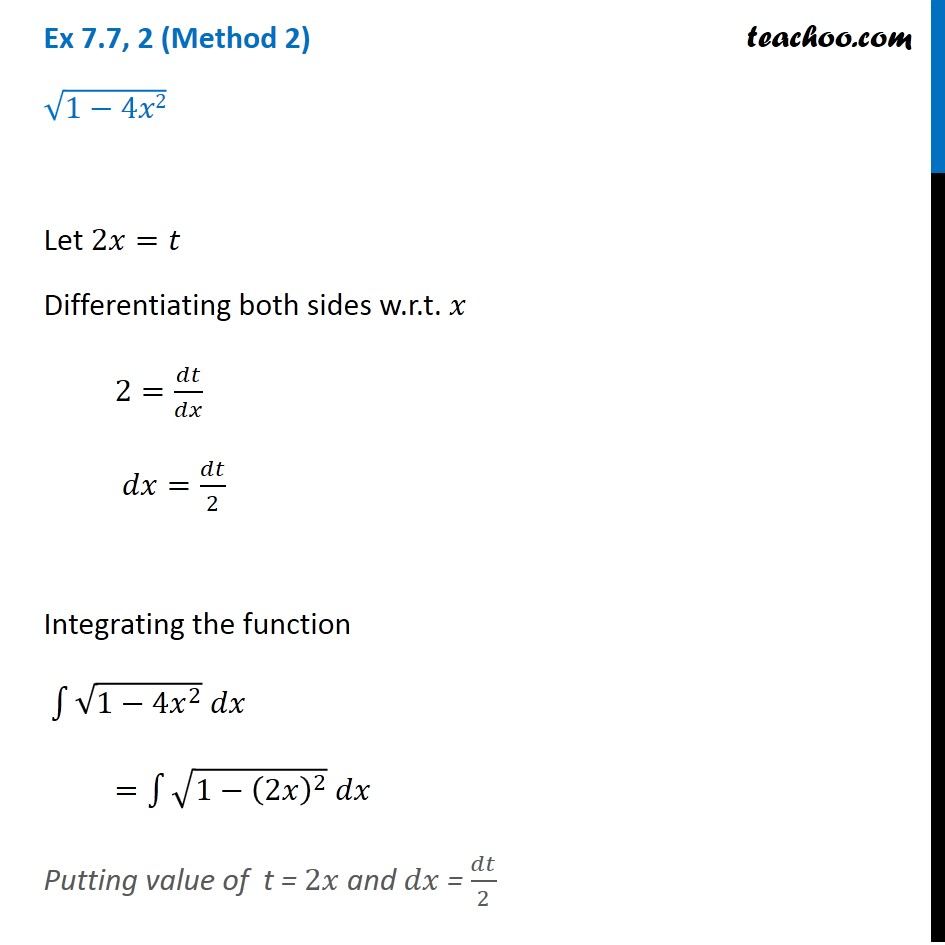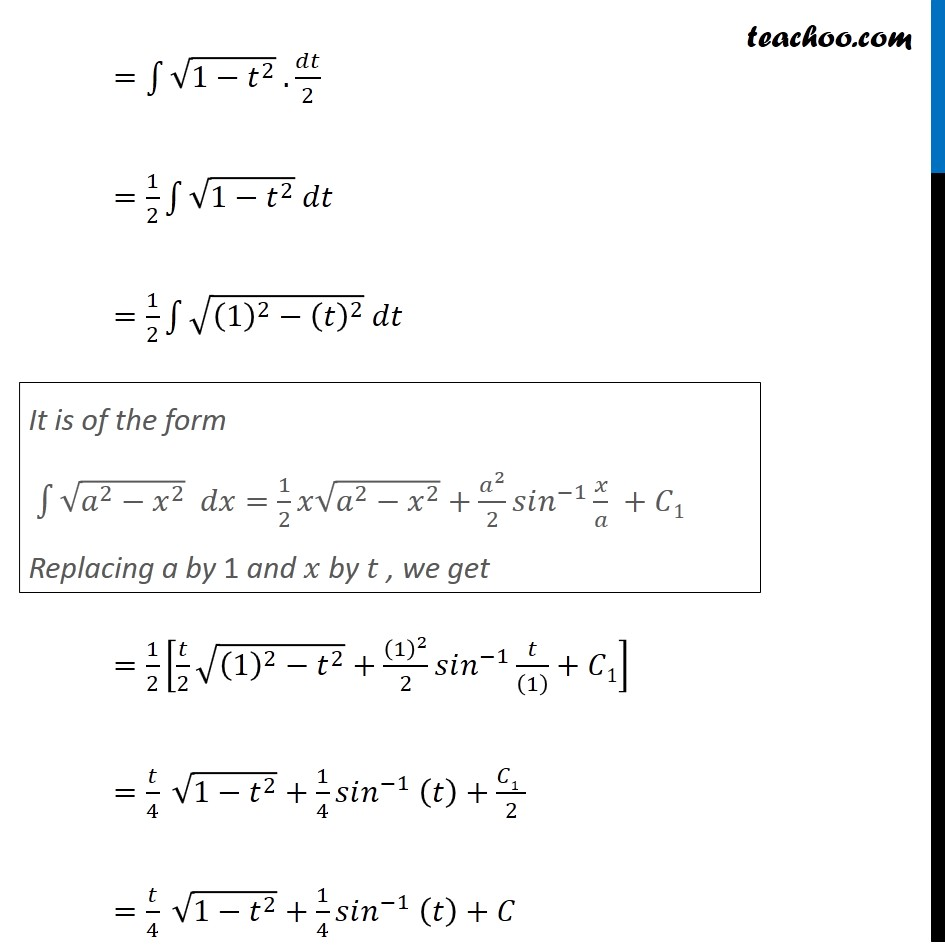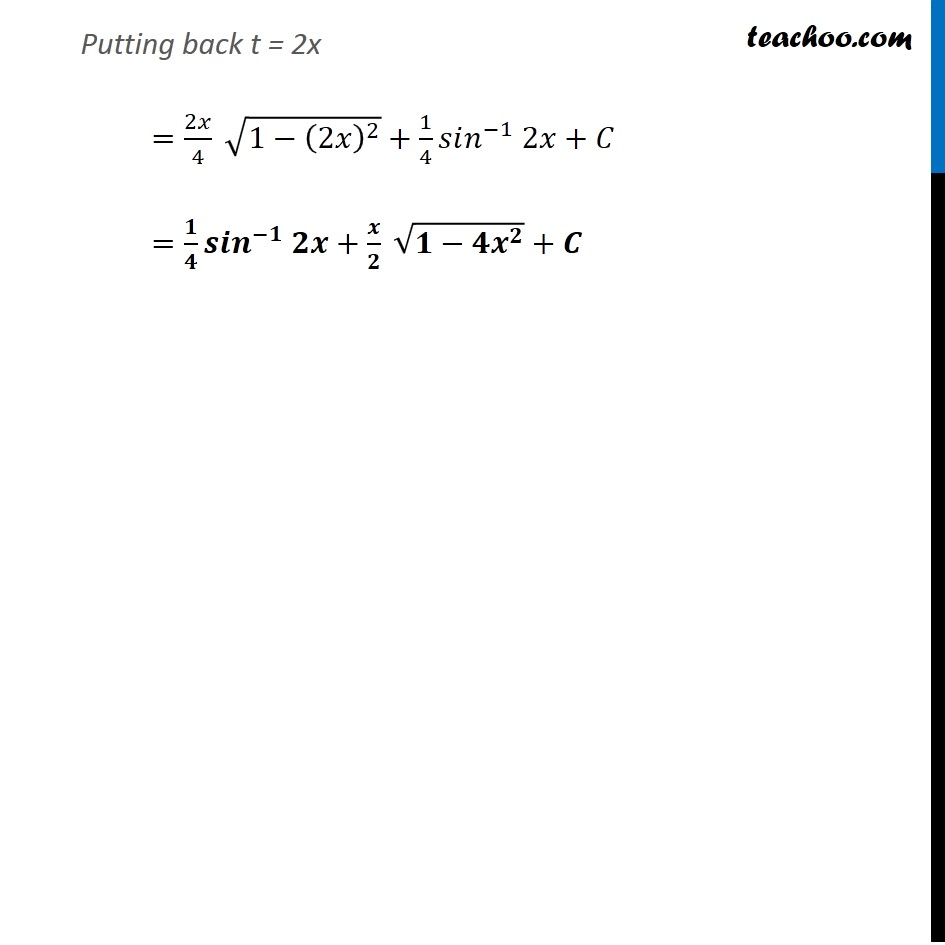Learn in your speed, with individual attention - Teachoo Maths 1-on-1 Class

### Transcript

Ex 7.7, 2 (Method 1) √(1−4𝑥2) ∫1▒〖√(1−4𝑥^2 ).𝑑𝑥〗 =∫1▒〖√(4(1/4−𝑥^2 ) ).𝑑𝑥〗 =∫1▒〖√4 √(1/4−𝑥^2 ).𝑑𝑥〗 =2∫1▒〖√((1/2)^2−𝑥^2 ).𝑑𝑥" " 〗 It is of the form ∫1▒〖√(𝑎^2−𝑥^2 ) .𝑑𝑥=1/2 𝑥√(𝑎^2−𝑥^2 )+𝑎^2/2 𝑠𝑖𝑛^(−1) 𝑥/𝑎+𝐶1〗 Replacing a with 2 and 1/2 , we get =2[1/2 𝑥√((1/2)^2−𝑥^2 ) +(1/2)^2.1/2 𝑠𝑖𝑛^(−1) 𝑥/(1/2)+𝐶1] =2[1/2 𝑥√(1/4−𝑥^2 ) +1/4 . 1/2 𝑠𝑖𝑛^(−1) 2𝑥+𝐶1] =𝑥√(1/4−𝑥^2 )+1/4 𝑠𝑖𝑛^(−1) 2𝑥+2𝐶1 =1/4 𝑠𝑖𝑛^(−1) 2𝑥+𝑥√((1 − 4𝑥^2)/4)+𝐶 =𝟏/𝟒 𝒔𝒊𝒏^(−𝟏) 𝟐𝒙+𝟏/𝟐 𝒙√(𝟏 − 𝟒𝒙^𝟐 )++𝑪 [where 𝐶=2𝐶1] Ex 7.7, 2 (Method 2) √(1−4𝑥2) Let 2𝑥=𝑡 Differentiating both sides w.r.t. 𝑥 2=𝑑𝑡/𝑑𝑥 𝑑𝑥=𝑑𝑡/2 Integrating the function ∫1▒〖√(1−4𝑥^2 ) 𝑑𝑥〗 =∫1▒〖√(1−(2𝑥)^2 ) 𝑑𝑥〗 Putting value of t = 2𝑥 and 𝑑𝑥 = 𝑑𝑡/2 =∫1▒〖√(1−𝑡^2 ) .𝑑𝑡/2〗 =1/2 ∫1▒〖√(1−𝑡^2 ) 𝑑𝑡〗 =1/2 ∫1▒〖√((1)^2−(𝑡)^2 ) 𝑑𝑡〗 =1/2 [𝑡/2 √((1)^2−𝑡^2 )+(1)^2/2 𝑠𝑖𝑛^(−1) 𝑡/((1) )+𝐶1] =𝑡/4 √(1−𝑡^2 )+1/4 𝑠𝑖𝑛^(−1) (𝑡)+𝐶1" " /2 =𝑡/4 √(1−𝑡^2 )+1/4 𝑠𝑖𝑛^(−1) (𝑡)+𝐶 It is of the form ∫1▒〖√(𝑎^2−𝑥^2 ) 𝑑𝑥=1/2 𝑥√(𝑎^2−𝑥^2 )+𝑎^2/2 𝑠𝑖𝑛^(−1) 𝑥/𝑎 +𝐶1〗 Replacing a by 1 and 𝑥 by 𝑡 , we get Putting back t = 2x =2𝑥/4 √(1−(2𝑥)^2 )+1/4 𝑠𝑖𝑛^(−1) 2𝑥+𝐶 =𝟏/𝟒 𝒔𝒊𝒏^(−𝟏) 𝟐𝒙+𝒙/𝟐 √(𝟏−𝟒𝒙^𝟐 )+𝑪